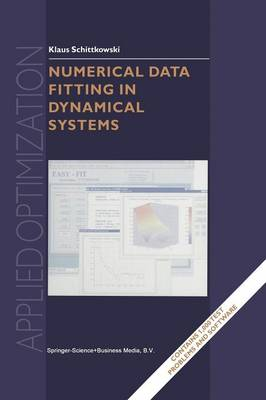Days : Hours : Minutes : Seconds•# Numerical Data Fitting in Dynamical Systems: A Practical Introduction with Applications and Software - Applied Optimization 77 (Paperback)

(author)
£224.50
Paperback 396 Pages / Published: 26/04/2013
• We can order this

Usually dispatched within 3 weeks

Real life phenomena in engineering, natural, or medical sciences are often described by a mathematical model with the goal to analyze numerically the behaviour of the system. Advantages of mathematical models are their cheap availability, the possibility of studying extreme situations that cannot be handled by experiments, or of simulating real systems during the design phase before constructing a first prototype. Moreover, they serve to verify decisions, to avoid expensive and time consuming experimental tests, to analyze, understand, and explain the behaviour of systems, or to optimize design and production. As soon as a mathematical model contains differential dependencies from an additional parameter, typically the time, we call it a dynamical model. There are two key questions always arising in a practical environment: 1 Is the mathematical model correct? 2 How can I quantify model parameters that cannot be measured directly? In principle, both questions are easily answered as soon as some experimental data are available. The idea is to compare measured data with predicted model function values and to minimize the differences over the whole parameter space. We have to reject a model if we are unable to find a reasonably accurate fit. To summarize, parameter estimation or data fitting, respectively, is extremely important in all practical situations, where a mathematical model and corresponding experimental data are available to describe the behaviour of a dynamical system.

Publisher: Springer-Verlag New York Inc.
ISBN: 9781475760507
Number of pages: 396
Weight: 629 g
Dimensions: 235 x 155 x 21 mm
Edition: Softcover reprint of the original 1st ed. 200